﻿ Single Arm, Centrifugal, Water Turbine for Low Head and Low Flow Application: Part 2- Performance EvaluationEnergy and Power

p-ISSN: 2163-159X    e-ISSN: 2163-1603

2018;  8(2): 56-60

doi:10.5923/j.ep.20180802.04### Single Arm, Centrifugal, Water Turbine for Low Head and Low Flow Application: Part 2- Performance Evaluation

Kiplangat C. Kononden1, Augustine B. Makokha1, Sitters W. M. Cox2

1Department of Energy Engineering, Moi University, Eldoret, Kenya

2Department of Civil & Structural Engineering, Moi University, Eldoret, Kenya

Correspondence to: Augustine B. Makokha, Department of Energy Engineering, Moi University, Eldoret, Kenya.
 Email: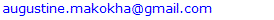Abstract

This paper presents performance analysis of the single arm centrifugal reaction water turbine described in Part 1 of our research. Experimental tests were conducted to assess the power output and efficiency of the turbine by varying the head, orifice diameter and the arm radius. The results obtained show that; the radial arm length affects the operating angular velocity, output torque as well as the overall turbine efficiency. The turbine exhibited maximum efficiency of 83% and 40% at heads of 1.85m and 11.5m respectively. The corresponding ratio of cross-sectional area of nozzle to main conduit was in the range of 0.18 to 0.24. It is concluded that the semi-circular centrifugal reaction water turbine is a fairly suitable type of turbine for low head and low-medium flow application. Based on the results obtained, an arm conduit with a larger cross-sectional area would be recommendable to achieve higher discharge. But further research is still needed on how best the water from the reservoir would get into the turbine and exit.

Keywords: Turbine, Low head, Low flow, Centrifugal, Micro-hydro

Cite this paper: Kiplangat C. Kononden, Augustine B. Makokha, Sitters W. M. Cox, Single Arm, Centrifugal, Water Turbine for Low Head and Low Flow Application: Part 2- Performance Evaluation, Energy and Power, Vol. 8 No. 2, 2018, pp. 56-60. doi: 10.5923/j.ep.20180802.04.

### 1. Introduction

Hydropower is a very good example of renewable energy and it is the most reliable base-load energy source. Nevertheless, there is a huge untapped exploitable hydro energy potential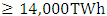[1, 2] mainly from Micro-hydropower sources waiting to be utilized throughout the world.
There are few small to medium scale low head and low volume flow rate hydro turbines commercially available, but they are very expensive and inefficient for micro power generation at individual level. Therefore, there is an inherent need of a simple, efficient and low cost hydro turbine that can enhance the exploitation of hydropower available in small rivers and streams where the head and flow rate are typically low.
Past research on simple reaction turbine [3, 4] show that fluid frictional losses are mainly dependent on the exit velocity (relative velocity) of the water leaving the turbine. In his research Date  managed to build a simple reaction turbine prototype that performed with a maximum efficiency of 66.7% while operating with a head of 4.5 and a flow rate of 21L/sec (0.021m3/sec). However the effect of turbine structure on the turbine’s performance as well as effect of the operating head is still not well understood . Moreover, the current knowledge on the effect of orifice variation on the turbine performance is not sufficient for absolute conclusions.
This work extends on other experiments in the field of simple reaction turbines with the aim of carrying out a comprehensive performance analysis of the single arm centrifugal reaction water turbine. This would yield more information on nozzle size variation, head variation as well radial arm length variation and their optimums in relation to turbine performance.

### 2. Experimental Work

#### 2.1. Methodology

The model turbine utilised in this study was fabricated at the University’s Engineering workshop using the locally available materials. Design details of the turbine can be reviewed in Part 1 of our work. The design parameters that were varied included; nozzle size, radial arm length and the supply head.
The ratio of cross-sectional area of main pipe to nozzle is another key design parameter that was assessed to establish the optimum ratio. A set of nozzle sizes were randomly selected starting from the highest to the smallest while maintaining an even distribution of the same. The ratios investigated were ≤ 1. A ratio of one represent a case where no nozzle is used (i.e. orifice diameter is equal to the main conduit internal diameter), while a ratio of zero represent a case where the turbine conduit has no exit (i.e. flow is zero). The internal diameter of the main radial conduit was 11.5mm, while the nozzle diameters were; 8.8mm, 6.0mm, 3.8mm, 2.5mm. In order to get the nozzle to main pipe cross-sectional area ratio, the cross-sectional area of the orifice of the nozzle is divided by cross-sectional area of the main pipe and is denoted as (1/n). For the nozzle diameters selected here, the ratio (1/n) equals; 1, 0.5856, 0.2722, 0.1092, 0.0473, 0 arranged from the highest to the lowest.
The arm radius was varied in three steps of R = 0.25m, 0.5m, 1.0m. By varying the radial arm length and keeping other variables constant and measuring the variations in the angular speed, torque and flow rate, the effects of the radial arm length on the output power were studied.
To study the effect of head variation the experiments were performed under two different heads of H = 1.85m and 11.5m. For each experiment the efficiency of the turbine was calculated. The lower head of H=1.85m was set by connecting the delivery pipe to the raised tank. Conversely the high head of H=11.5m was achieved by directly connecting the supply pipe to the high pressure tap source at the Laboratory.

#### 2.2. Experimental Setup

Figure 1 shows a snap shot of the setup of the turbine test rig used in the current study. The test rig consists of the following components;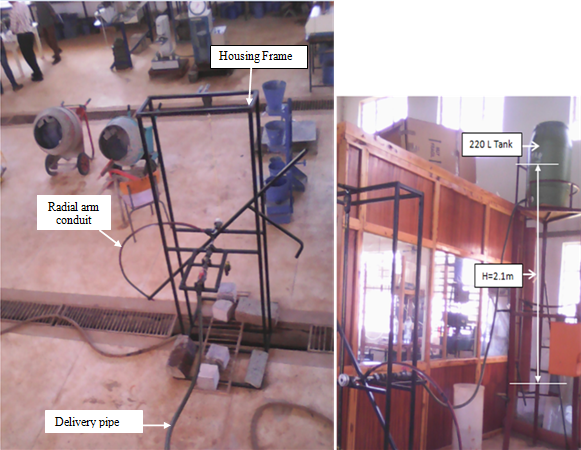Figure 1. Picture of the turbine set up
× Hydraulic power input components comprising the raised water storage tank (tank capacity 0.22m³), high pressure water tap, supply pipe, delivery pipe (penstock), and flow control valve.
× Flow meter and pressure gauge used for input power measurement.
× Power output components that encompass the turbine housing frame, single arm centrifugal reaction water turbine with the inlet rotary lip sill joint arrangement.
× Tachometer (for angular speed measurement)
The turbine shaft torque is measured using a rope brake dynamometer. The output power is obtained by the product of torque and angular speed. The digital tachometer is attached to the turbine’s shaft so as to measure the angular speed.
Figure 2 is a schematic diagram of the turbine test rig which shows the position and arrangement of; raised water storage tank, high pressure water tap, supply pipe, delivery pipe (penstock), and flow control valve.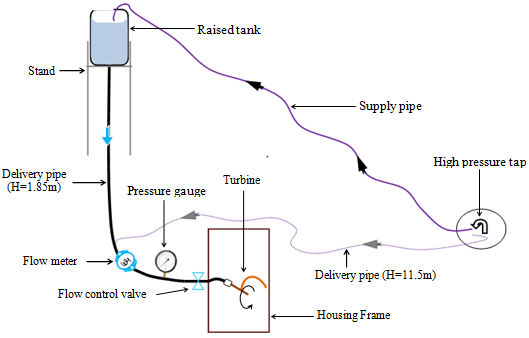Figure 2. Schematic diagram of the test rig
Figure 3 shows the various components that were used to set up a rope brake dynamometer.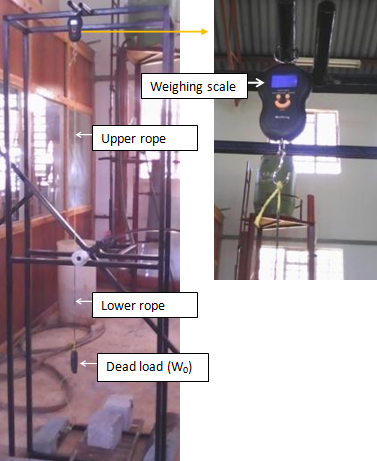Figure 3. Rope Brake dynamometer with a digital weighing machine

#### 2.3. Test Procedure

In all the experimental tests conducted both the input and output power tests are done simultaneously. At the start of the tests, the turbine is allowed to rotate freely without any load, while the operating head is kept constant. Using the relevant instruments, the parameters such as flow rate, rotational speed and operating torque were measured simultaneously and recorded. A dead load was placed on the lower rope of the rope brake dynamometer as shown in figure 3 and is gradually increased in steps, leading to a decrease in the rotational speed of the turbine. The decrease in rotational speed tends to penalize the centrifugal pumping effect causing a reduction in the water flow rate. Note that, the flow rate, rotational speed, and operating torque are recorded each time the load is increased. The increase in load is maintained till the turbine slows down to a stop. The experiment is repeated for each radial arm length and for the five nozzle sizes.
This procedure is replicated for the higher operating head of 11.5m. This was done for purposes of comparing the performance characteristics of the turbine under low hydrostatic head (H=1.85m) and under high hydrostatic head (H=11.5m). All the generated data were recorded manually on data charts. The obtained data was analysed using Microsoft Excel.

### 3. Results and Discussion

#### 3.1. Turbine Efficiency

From theoretical analysis, turbine efficiency is given by the following relation;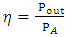(1)
Where,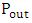is the theoretical output power while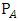is the available power. They are obtained from the following relations: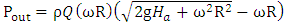(2)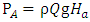(3)
Where Q is the flowrate,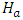is the actual operating height after subtracting all the head losses. The other parameters retain their previous definitions. Using equation (2) and (3), the theoretical output poweris obtained as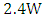while the available poweris obtained as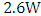. This gives theoretical efficiency of 93.4%. The values of parameters used in the calculations are as follows: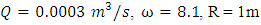and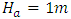. The value of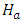is obtained using the relation,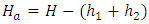in which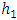and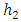are the average head losses due to frictional resistance in the delivery pipe and the turbine’s semicircular pipe, computed using Darcy-Weisbach equation .

#### 3.2. Nozzle Size and Arm Length

The trends in turbine efficiency for different values of nozzle to main pipe cross-sectional area ratio (1/n) and arm length (R) were studied to find the optimum levels. The highest efficiencies for each of the five nozzles used in the low head and high head experiments were compared. The procedure was replicated for each of the three arm lengths.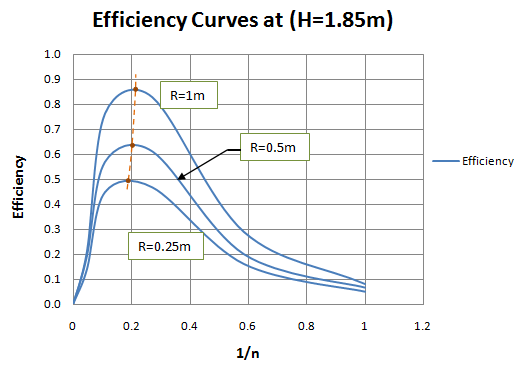Figure 4. Efficiency against (1/n) for different arm radii when H=1.85m
From the results in Figure 4, at peak efficiency increases with increase in arm length, R. At low arm radius the efficiency is low but as the radius increase the efficiency also increases. This is because with lower arm radius the available torque is barely enough to overcome the frictional torque due to the weight of the entire turbine and other dynamic imbalances.
The optimum ‘nozzle to main pipe cross-sectional area ratio’ (1/n) is observed to be approximately 0.22 corresponding to arm radius of R=1m. In the case of R = 0.5m, the optimum ratio (1/n) is at 0.2, whereas in the case of R = 0.25m, the optimum ratio (1/n) occurs at 0.18.
Figure 5 shows the performance characteristics under a higher head of 11.5m. As seen from the figure the optimum ‘nozzle to main pipe cross-sectional area ratio’ (1/n) is 0.24 for arm radius R = 1m, 0.22 for arm radius R = 0.5m and 0.2 for arm radius R = 0.25m.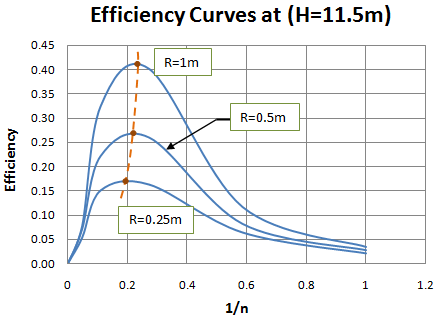Figure 5. Efficiency against (1/n) for different arm radii at H=11.5m
From the results presented, the optimum ‘nozzle to main pipe cross-sectional area ratio’ that corresponds to the highest efficiency for a particular set-up is determined. The optimum efficiency of the turbine reduces with decrease in the arm radius. Reduction in arm radius leads to reduction in the operating torque. Low operating torque accounts for low turbine efficiency. However with the decrease in the arm radius it means that there is a reduction in frictional head losses.
The reason for low efficiencies at higher (1/n) ratios is attributable to high resistance at the nozzle area. Also it is possible that the ratio of the resistive bearing frictional torque to the driving torque is high.
The observed trends imply that not all nozzles give the same torque or the same angular speed given relatively equal potential energy.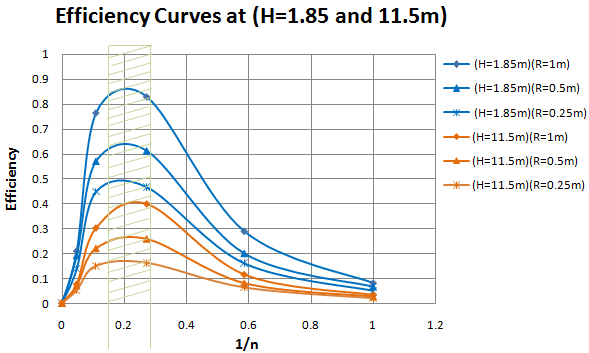Figure 6. Efficiency against (1/n) for low and high head
Figure 6 combines the results of figures 4 and 5. It is evident that efficiency is high when operating at a relatively lower head than at high head. This can be explained mathematically by equation (4);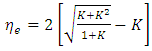(4)
Where, ‘k’ is a proportionality factor depending on the roughness and other losses in the nozzle. Conversely parameter ‘K’ is used to denote a non-dimensional factor representing the ratio of the head due to angular acceleration to the head due to gravitational acceleration as shown in equation (5).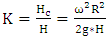(5)
This therefore means that, at a particular optimum angular velocity and radius, the higher the operating head the lower the value of K which translates to lower operating efficiency.

#### 3.3. Optimum Values of Parameter ‘K’ and Factor ‘k’

Using the calculated theoretical efficiency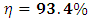, as well as the actual experimental efficiency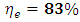, the values of parameters ‘K’ and ‘k’ can be obtained. From the theoretical analysis of the turbine the expression of theoretical efficiency is given by equation (6);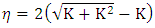(6)
Using equation (6), K is found to be 3.30.
Using the obtained value of K = 3.30 and applying equation (4) the value of factor k is obtained as 0.028.

### 4. Conclusions

The main objective of this study was to assess the performance of a model scale single arm centrifugal water turbine in terms of efficiency and power. The primary design parameters were nozzle size and arm length while operational parameters were flow rate, available head and rotational speed. The results show that, the turbine efficiency increases with increase in arm radius for the range tested here. The maximum turbine efficiency obtained at low head (H=1.85m) was 83%, and at high head (H=11.5m) was 40.11%. This implies that a lot of potential energy is wasted when operating at relatively higher heights for this type of turbine. Further, the results reveal that an optimum operating nozzle to main conduit cross-sectional area ratio lies between 0.18 and 0.24. These results reinforce findings by other researchers that a single arm centrifugal reaction water turbine is economical for low head and low flow conditions. Nonetheless, further research is needed to establish the most suitable inlet and discharge mechanism for the turbine.

### ACKNOWLEDGEMENTS

The authors wish to thank the Centre of Excellence in Phytochemistry, Textiles and Renewable Energy (ACE II) at Moi University for sponsoring this research and the technical staff of mechanical engineering workshop for their help during the fabrication of the turbine prototypes and test rig modification.

### References

  R. Taylor, “Hydropower,” In: A. C. J Trinnaman, Ed., Survey of Energy Resources, Elsevier Science, Oxford, 2004, pp. 199-232.  G. L. Sommers, “Appendix 4: Selected Energy-Related Tables,” In: C. J. Cleveland, Ed., Encyclopedia of Energy, Elsevier, New York, 2004, pp. 721-775.  A. Akbarzadeh, C. Dixon and P. Johnson, “Parametric Analysis of a Simple Reaction Water Turbine and Its Application for Power Production from Low Head Reservoirs,” Proceedings of Fluids Engineering Division Summer Meeting, New Orleans, 29 May-1 June 2001.  A. Date and A. Akbarzadeh, “Design and Cost Analysis of Low Head Simple Reaction Hydro Turbine for Remote Area Power Supply,” Renewable Energy, Vol. 34, No. 2, 2009, pp. 409-415.  Date, Abhijit, Ashwin Date, and Aliakbar Akbarzadeh. "Performance investigation of a simple reaction water turbine for power generation from low head micro hydro resources." Smart Grid and Renewable Energy 3.03 (2012): 239.  Calvert J. B. (n.d.). Turbines. Retrieved September 12, 2015, from https://mysite.du.edu/~jcalvert/tech/fluids/turbine.htm History and Modeling of turbines.  Garshelis, Ivan J. "Torque and Power Measurement." The Mechatronics Handbook, -2 Volume Set 19 (2002): 48.  Merle, C. P and David, C. W. Mechanics of Fluids, 2nd ed. Prentice Hall, USA, 1997.